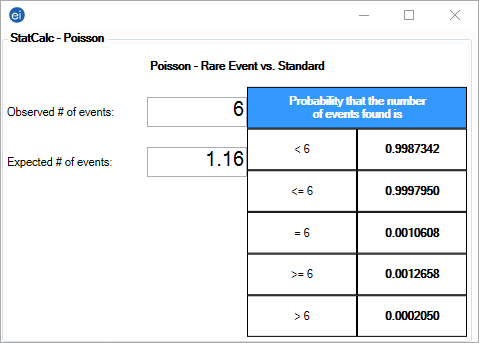# StatCalc: Statistical Calculators

### Poisson

To analyze the Poisson distribution, enter the expected number of positive outcomes in Expected # of events and the value of positive outcomes you would like to determine the probability of in Observed # of events.Figure 10.14: Poisson Distribution

The results show that there is a 0.13% chance of having six or more positive outcomes when the expected value is 1.16. Additional percentages are stated for the ranges outlined in the left column of the table.

Page last reviewed: September 16, 2022, 12:00 pm